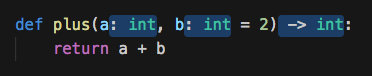Ocavue's Blog
Python 中的类型标注 2018-12-31

## # Python type hints 的进化

### # Python 3.0: function annotation

``````def plus(a, b=1):
return a + b
``````

``````def plus(a: int, b: int = 2) -> int:
return a + b
``````Python 解释器在运行时并不会检查类型，所以哪怕参数的类型不对，Python 解释器也不会因此抛出任何异常。

``````# example.py
def plus(a: int, b: int = 2) -> int:
return a + b

print(plus("hello ", "world!"))
``````
``````\$ python3 example.py
hello world!
``````

``````\$ pip3 install mypy
``````

``````\$ mypy example.py
example.py:5: error: Argument 2 to "exp" has incompatible type "str"; expected "int"
``````

``````from .mypackage import Bar

def f(bar: Bar) -> None:
print(bar.bar_method())
``````

``````\$ pyenv local python3.0.1; python
Python 3.0.1 (r301:69556, May 24 2018, 14:39:16)

>>> def f(a: int, b: str): pass
...
>>>
``````

### # Python 3.5: typing

``````from typing import Dict, List

def best_students(scores: Dict[str, int]):
return {name: score for name, score in scores.items if score >= 90}
``````

`Dict[str, int]` 表示一个 keys 的类型为 str，values 的类型为 int 的字典，比如 `{"a": 1, "b": 2}`

`List[int]` 表示由整型组成的列表，比如`[0, 1, 1, 2, 3]`

`Dict[str, Dict[str, List[str]]]`

``````{
'原木镇': {
'第一小学': ['张伟', '王伟', '王芳'],
'第二小学': ['李伟', '李娜'],
},
'鸽子镇': {
'高山中学': ['张敏', '李静'],
'亿百中学': ['王静']
'蟒蛇小学': ['刘伟', '王秀英']
}
}
``````

## # typing 高级用法

### # Union

``````from typing import Union, List

def get_first_name(names: List[str]) -> Union[None, str]:
if len(names) >= 1:
return names
else:
return None
``````

### # Callable

Python 中万物皆是对象，函数也是对象。`Callable` 就可以表示函数类型。

``````from typing import Callable

def get_regex() -> Callable[[str, str], bool]:
def regex(pattern: str, string: str) -> bool:
return re
return regex()

``````

### # Any

Python 总有一些我们很难表达的形式或者类型。这种情况下我就能使用 `typing.Any`，它代表任何东西。比如说

``````from typing import Any

def log(msg: Any):
print(Any)
``````

### # Python 3.6: variable annotations

``````items: list = read_json_file()
``````
``````class Employee(NamedTuple):
name: str
id: int = 3
``````

### # Python 3.7: dataclass

``````>>> def foo(a: str):
...     print('hello', a)
...

>>> foo.__annotations__
{'a': str}

>>> class Bar:
...     a: str
...     b: int

>>> Bar.__annotations__
{'a': str, 'b': int}
``````

``````from typing import Tuple

class Bar:
def __init__(
self, name: str, size: float, phone: int, location: Tuple[float, float]
):
self.name = name
self.size = size
self.phone = phone
self.location = location
``````

``````from dataclasses import dataclass
from typing import Tuple

class Bar(dataclass):
name: str
size: float
phone: int
location: Tuple[float, float]
``````

dataclass 作为一个例子，展示了 Python 的 type hints 的潜力。

## # python typing 不能做什么

There is no syntax to indicate optional or keyword arguments; such function types are rarely used as callback types. `Callable[..., ReturnType]` (literal ellipsis) can be used to type hint a callable taking any number of arguments and returning `ReturnType`.

``````def foo(item: str) -> None:
print(item + "s")

foo(item=0)
``````

``````\$ mypy example.py
example.py:5: error: Argument "item" to "foo" has incompatible type "int"; expected "str"
``````

``````import time
from typing import Callable

def foo_timer(func) -> Callable[..., None]:
if __debug__:

def new_func(*args, **kwargs) -> None:
start = time.time()
result = func(*args, **kwargs)
print(time.time() - start)
return result

return new_func
else:
return func

@foo_timer
def foo(*, item: str) -> None:
print(item + "s")

foo(item=0)
``````
``````\$ mypy example.py
\$
``````